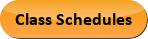Sep 21, 2023
2022-2023 College Catalog
 Select a Catalog 2023-2024 College Catalog 2022-2023 College Catalog [ARCHIVED CATALOG] 2021-2022 College Catalog [ARCHIVED CATALOG] 2020-2021 College Catalog [ARCHIVED CATALOG] 2019-2020 College Catalog [ARCHIVED CATALOG] *** DRAFT 2024-2025 College Catalog DRAFT *** [ARCHIVED CATALOG]
 HELP 2022-2023 College Catalog [ARCHIVED CATALOG] Print-Friendly Page (opens a new window) Add to Portfolio (opens a new window)

# MAT 092 - Elementary Algebra

3 Credits, 3 Contact Hours
3 lecture periods 0 lab periods

Includes translating written statements into algebraic expressions, solving linear equations and inequalities, graphing linear equations, and solving systems of equations in two or three variables. Also includes integer exponents, scientific notation, polynomial operations, and factoring of polynomials.

Prerequisite(s): Within the last three years: ICS 081  with a grade of B or better, or MAT 086 with a grade of C or better, or completion of module 15 in MAT 089A  or MAT 089B , or satisfactory score on the Mathematics assessment test.
Corequisite(s): MAT 092LBCourse Learning Outcomes
1. Solve multi-step linear equations including decimal and fractional coefficients and solutions.
2. Graph linear equations using a variety of techniques.
3. Solve linear systems using graphical and algebraic techniques.
4. Factor polynomials using a variety of techniques.

Performance Objectives:
1. Express written statements algebraically.
2. Solve linear equations.
3. Solve linear inequalities and graph solutions on the number line.
4. Graph linear equations.
5. Solve systems of linear equations in two variables graphically and algebraically.
6. Solve systems of linear equations in three variables algebraically.
7. Apply the laws of exponents to expressions with integer exponents.
8. Perform the basic operations on polynomials.
9. Factor polynomials using multiple techniques.

Optional Objectives:

1. Simplify, add, subtract, multiply, and divide elementary rational expressions; solve rational equations that result in linear equations.
2. Simplify numerical square-roots.
3. Solve quadratic equations by factoring, taking square roots, and the quadratic formula

Outline:
1. Translate Written Statements Into Algebraic Expressions
2. Linear Equations
1. Simplify and solve algebraically
2. Applications
3. Linear Inequalities
1. Solve
2. Graph on the number line
3. Applications
4. Lines
1. Cartesian coordinate system
2. Midpoint of a line segment
3. Slope and intercepts
4. Equations
1. Vertical and horizontal
2.  Standard form
3.  Slope-intercept form
4.  Point-slope form
5. Parallel and perpendicular
5. Systems of Linear Equations in Two Variables
1. Graphing method
2. Substitution method
3. Elimination method
4. Applications
6. Systems of Linear Equations in Three Variables
1. Substitution method
2. Elimination method
3. Applications
7. Integer Exponents
1. Laws of exponents
2. Negative exponents
3. Scientific notation
8. Polynomials
1. Terminology
2. Operations including addition, subtraction, multiplication, and division (including long division)
9. Factoring Polynomials
1. Greatest common factor
2. Factor by grouping
3. Trinomials
4. Difference of squares
5. Difference/sum of cubes
10. Optional Topics
1. Elementary Rational Expressions
1. Avoid division by zero
2. Simplification
3. Basic operations
4. Solve rational equations which result in linear equations
5. Applications
2. Square Roots
1. Evaluate
2. Simplify
3. Solve radical equations which result in linear equations